Cornell Math Explorers' Club Chaos and FractalsMath Explorers' ClubIntroduction to ChaosOne-Dimensional MapsThe Feigenbaum MapThe Chaos GameThe Mandelbrot SetConway's Game of Life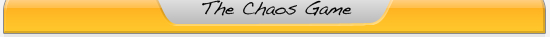# The Chaos Game

Each student (and teacher, if there are not many students) gets the following:

• an overhead transparency with the vertices of a triangle colored 3 different colors (red, orange, and green)
• a die with each of the 3 colors on 2 of its sides
• a transparency marker pen
• a paper ruler
• a temporary marker (a little circle from a hole-punch or just a small scrap of paper)

The game is played as follows:

1. Select a seed (initial) point (preferably within the vertices of the triangle) using the temporary marker.
2. Roll the die and move the temporary marker half the distance to the vertex of the same color of the roll.
3. Repeat this between 7 and 10 times with the temporary marker.
4. For the next 20 to 30 rolls keep track of the orbit using the marker pen.

A good question for the students at this point - "What do you expect the orbits to look like?" Some will probably say a random display of points. Others may expect the points to fill up the triangle (and they may say Sierpinski's Triangle).

Now collect all the transparencies (plus the supplied already-completed transparencies) and place them on top of one another (can use an overhead projector to show the students or simply have them gather around the teacher and hold them up to the light). Hopefully everyone calculated enough points and an image of Sierpinski's Triangle should appear. But what is Sierpinski's Triangle and why did it show up?

NOTE 1: If this part goes very quickly, the students can play the game again but this time move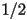the distance to the pink vertex,the distance to the green vertex, and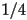the distance to the orange vertex. However, they can also do this using one of the computer programs as well so it's probably best to move on.

NOTE 2: The students caught on very quickly that it would take hundreds of points for a pattern to form. Therefore I included a bunch of pre-done Chaos Game boards just in case.

# The Sierpinski Triangle

The Sierpinski Triangle can also be constructed using a deterministic rather than a random algorithm.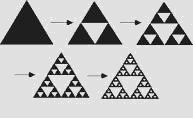At each stage of the iteration we form new equilateral triangles by connecting the midpoints of the sides of the triangles remaining from the previous iteration. Thus after 1 iteration the remaining triangles have dimensionsof the original triangles andthe area (this is easily proven). We now remove the newly formed "middle" triangle and leave 3 smaller triangles, each having dimensions(and area) of its "parent" triangle. We then repeat (or iterate) this process as desired. After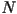iterations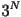triangles will remain, each have dimensions equal to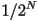of the original triangle's.

"One of the basic properties of fractal images is the notion of self-similarity."

~ Robert Devaney

The Sierpinski Triangle consists of 3 self-similar copies of itself, each with magnification factor 2. Therefore we can divide the fractal into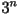congruent, self-similar pieces, each of which has dimensionsof the original triangle's.

What is the dimension of this object? Note that a line is 1-dimensional and a square is 2-dimensional (Do the students understand why? Both are self-similar.)

"The dimension is simply the exponent of the number of self-similar pieces with magnification factor N into which the figure may be broken."

~ Robert Devaney

Show how this equation works for the line, square, and cube.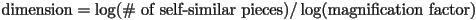We define the fractal (Hausdorff) dimension of a self-similar object to be:For the Sierpinski Triangle we have: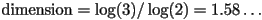Another example of a self-similar fractal is the von Koch curve: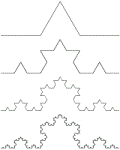The von Koch curve looks like it could be 1-dimensional, but it has infinite arc length and any 2 points on it are infinitely far away from one another. Its fractal dimension can be computed with the following equation: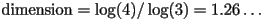It has fractal dimension: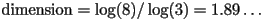Activity - Creating Fractals

There is a general way to recursively create many types of fractals, called fractal decomposition. One starts with a shape oriented in a space along with a replacement rule which is used to refine the picture. All of the fractals on this page can be created in this manner. With the Sierpinski triangle, the base shape is a triangle and the replacement rule is to remove the triangle and replace it with 3 triangles of half the size which share vertices and edges with the original triangle. Similarly, the base shape for the Sierpinski Carpet is a square, and the replacement rule is removing the square and replacing it with eight others, each one-third the size, and in a particular location relative to the parent square.

There are myriad other ways to make replacement rules. One is to not remove any shapes once they have been put down, but only to add on to what already exists. Another is to use different replacement rules with various probabilities. Try several of these out, and figure out what kinds of rules lead to beautiful designs.

What commonalities are there among all of these different rules for generating fractals? One important one is a geometric decrease in size. That is that objects only beget other objects that are proportionally smaller than they themselves are. This ensures several things. The first and most obvious is that the shapes thus created are finite in extent. It also ensures that only a finite number of replacements need to be completed to obtain a good picture of what the fractal looks like. A less obvious implication is that the figures look qualitatively the same on all scales (scale-invariance). What other commonalities are there between rules that make "nice-looking" shapes?

A fern is also a popular fractal to create using various types of replacement rules. Can you figure out approximately what the rules for creating the fractal fern at the top of this page are?Cornell University - Chris Lipa - lipa@math.cornell.edu